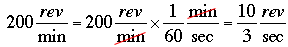SEARCH HOMEMath Central Quandaries & QueriesQuestion from Rebecca, a student: A point on a belt is moving at the rate of 30 ft/sec and the belt drives a pulley at the rate of 200 rpm. What is the radius of the pulley?Hi Rebecca,

First let's get the time units constant. I'm going to choose seconds. There are 60 seconds in a minute soThe circumference of a circle is 2 π times the radius so if the radius of the circle is r feet then its circumference is

2 πr feet.

The pulley moves 10/3 revolutions in a second so that's 2 πr × 10/3 feet in a second. But you are told that the belt, which runs on the pulley, moves at 30 feet in a second. Thus

30 = 2 πr × 10/3

Solve for r.

PennyMath Central is supported by the University of Regina and The Pacific Institute for the Mathematical Sciences.